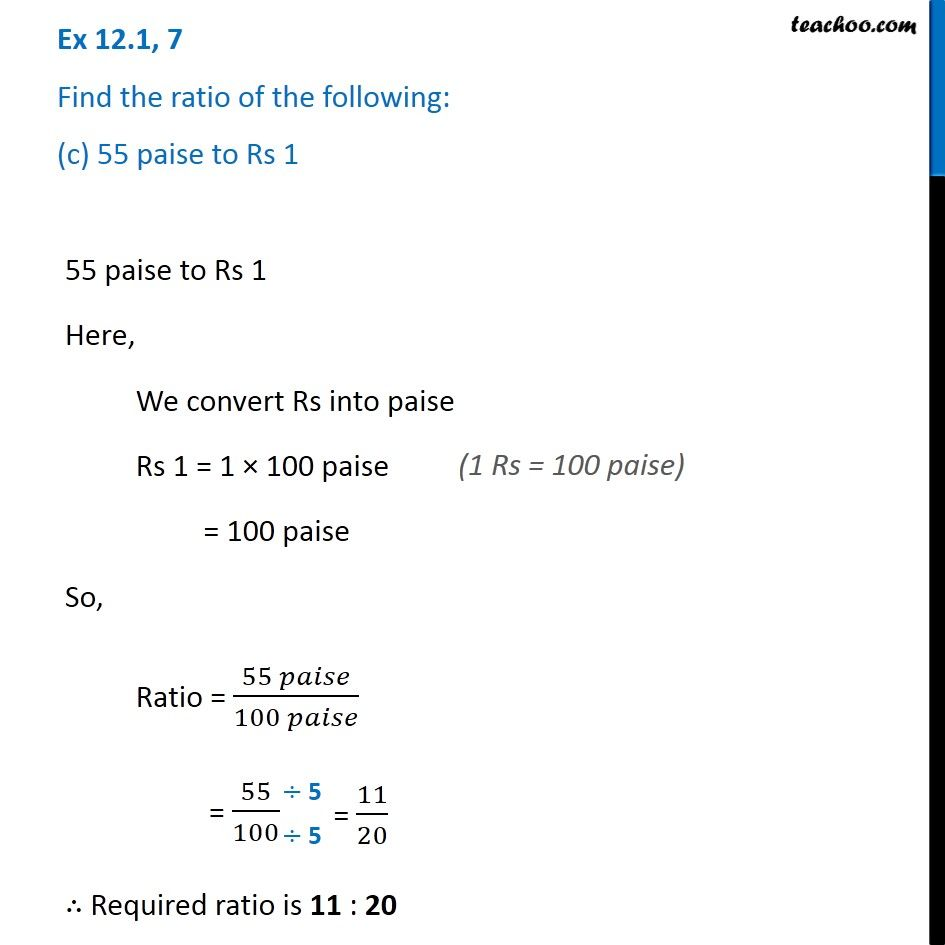Ex 12.1

Chapter 12 Class 6 Ratio and Proportion
Serial order wiseLearn in your speed, with individual attention - Teachoo Maths 1-on-1 Class

### Transcript

Ex 12.1, 7 Find the ratio of the following: (c) 55 paise to Rs 1 55 paise to Rs 1 Here, We convert Rs into paise Rs 1 = 1 × 100 paise = 100 paise So, Ratio = (55 𝑝𝑎𝑖𝑠𝑒)/(100 𝑝𝑎𝑖𝑠𝑒) = 55/100 ∴ Required ratio is 11 : 20